Electron. J. Differential Equations, Vol. 2018 (2018), No. 194, pp. 1-17.

### Positive solution for Henon type equations with critical Sobolev growth Kazune Takahashi

Abstract:
We investigate the Henon type equation involving the critical Sobolev exponent with Dirichret boundary condition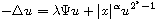in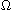included in a unit ball, under several conditions. Here,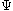is a non-trivial given function with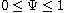which may vanish on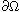. Let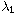be the first eigenvalue of the Dirichret eigenvalue problem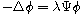in. We show that if the dimension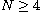and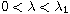, there exists a positive solution for small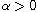. Our methods include the mountain pass theorem and the Talenti function.

Submitted April 2, 2018. Published November 28, 2018.
Math Subject Classifications: 35J20, 35J60, 35J61, 35J91.
Key Words: Critical Sobolev exponent; Henon equation; mountain pass theorem; Talenti function.

Show me the PDF file (279 KB), TEX file for this article.

 Kazune Takahashi Graduate School of Mathematical Sciences The University of Tokyo 3-8-1 Komaba Meguroku Tokyo 153-8914, Japan email: kazunetakahashi@gmail.com